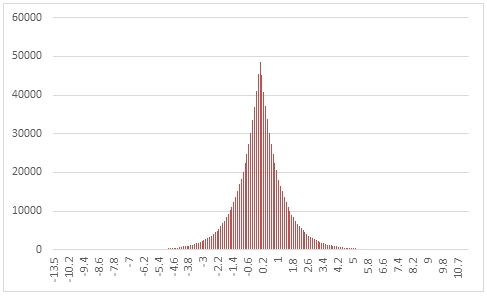# SQL Server random LaPlace distribution

RANDLAPLACE

Updated: 31 March 2014

Use the table-valued function RANDLAPLACE to generate a sequence of random numbers from a LaPlace distribution with parameters @Location and @Scale.
Syntax
SELECT * FROM [wctMath].[wct].[RANDLAPLACE](
<@Rows, int,>
,<@Location, float,>
,<@Scale, float,>)
Arguments
@Rows
the number of rows to generate. @Rows must be of the type int or of a type that implicitly converts to int.
@Location
the location parameter. @Shape must be of the type float or of a type that implicitly converts to float.
@Scale
the scale parameter. @Scale must be of the type float or of a type that implicitly converts to float.
Return Types
RETURNS TABLE (
[Seq] [int] NULL,
[X] [float] NULL
)
Remarks
·         @Scale must be greater than zero.
·         If @Shape is NULL then @Shape is set to 0.
·         If @Scale is NULL then @Scale is set to 1.
·         If @Rows is less than 1 then no rows are returned.
Examples
In this example we create a sequence 1,000,000 random numbers rounded to one decimal place from a Laplace distribution with @Location = 0 and @Scale =1, COUNT the results, paste then into Excel, and graph them.
SELECT
X,
COUNT(*) as [COUNT]
FROM (
SELECT
ROUND(X,1) as X
FROM
wct.RANDLAPLACE(
1000000, --@Rows
0,       --@Loations
1        --@Scale
)
)n
GROUP BY
X
ORDER BY
X
This produces the following result.In this example we generate 1,000,000 random numbers from a LaPlace distribution with @Shape of 5 and @Scale of 2. We calculate the mean, standard deviation, skewness, and excess kurtosis from the resultant table and compare th
ose values to the expected values for the distribution.
DECLARE @size as int = 1000000
DECLARE @location as float = -5
DECLARE @scale as float = 4
DECLARE @mean as float = @location
DECLARE @var as float = 2 * POWER(@scale,2)
DECLARE @stdev as float = SQRT(@var)
DECLARE @skew as float = 0
DECLARE @kurt as float = 3

SELECT
stat,
[RANDLAPLACE],
[EXPECTED]
FROM (
SELECT
x.*
FROM (
SELECT
AVG(x) as mean_LAPLACE,
STDEVP(x) as stdev_LAPLACE,
wct.SKEWNESS_P(x) as skew_LAPLACE,
wct.KURTOSIS_P(x) as kurt_LAPLACE
FROM
wct.RANDLAPLACE(@size,@location,@scale)
)n
CROSS APPLY(
VALUES
('RANDLAPLACE','avg', mean_LAPLACE),
('RANDLAPLACE','stdev', stdev_LAPLACE),
('RANDLAPLACE','skew', skew_LAPLACE),
('RANDLAPLACE','kurt', kurt_LAPLACE),
('EXPECTED','avg',@mean),
('EXPECTED','stdev',@stdev),
('EXPECTED','skew',@skew),
('EXPECTED','kurt',@kurt)
)x(fn_name,stat,val_stat)
)d
PIVOT(sum(val_stat) FOR fn_name in([RANDLAPLACE],[EXPECTED])) P

This produces the following result (your result will be different).

 stat RANDLAPLACE EXPECTED avg -5.00400757 -5 kurt 3.029291787 3 skew 0.017747871 0 stdev 5.668735766 5.656854249

### SupportCopyright 2008-2023 Westclintech LLC         Privacy Policy        Terms of Service